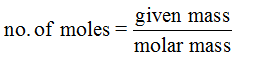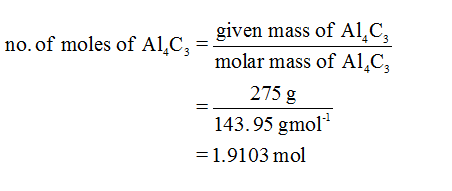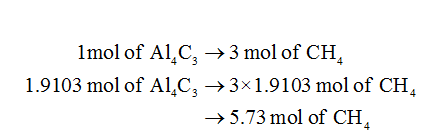# Given the equuation: Al4C3+12H2O=4Al(OH)3+3CH4How many moles of CH4 will be formed by the reaction of 275 grams of Al4C3 will excess water?

Question
25 views

Given the equuation: Al4C3+12H2O=4Al(OH)3+3CH4

How many moles of CH4 will be formed by the reaction of 275 grams of Al4C3 will excess water?

check_circle

Step 1

Mole is the amount of the substance that contains the same number of particles or atoms or molecules. Molar mass is defined as average mass of atoms present in the chemical formula. It is the sum of the atomic masses of all the atoms present in the chemical formula of any compound.Step 2

No. of moles of Al4C3 can be calculated is shown below:Step 3...

### Want to see the full answer?

See Solution

#### Want to see this answer and more?

Solutions are written by subject experts who are available 24/7. Questions are typically answered within 1 hour.*

See Solution
*Response times may vary by subject and question.
Tagged in